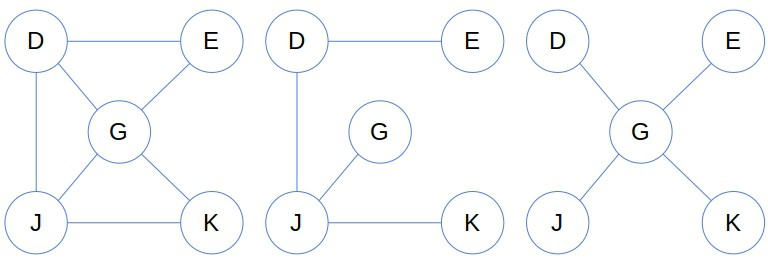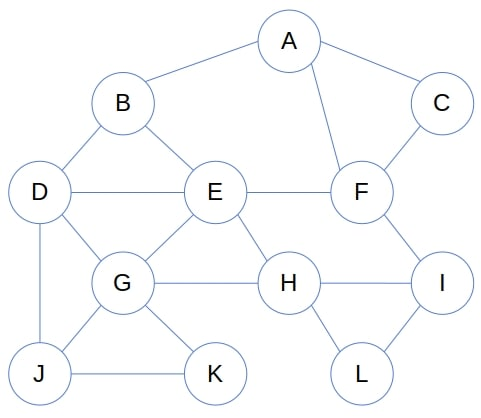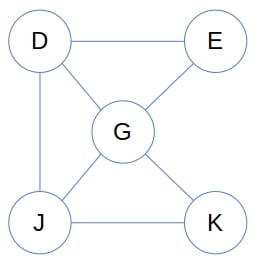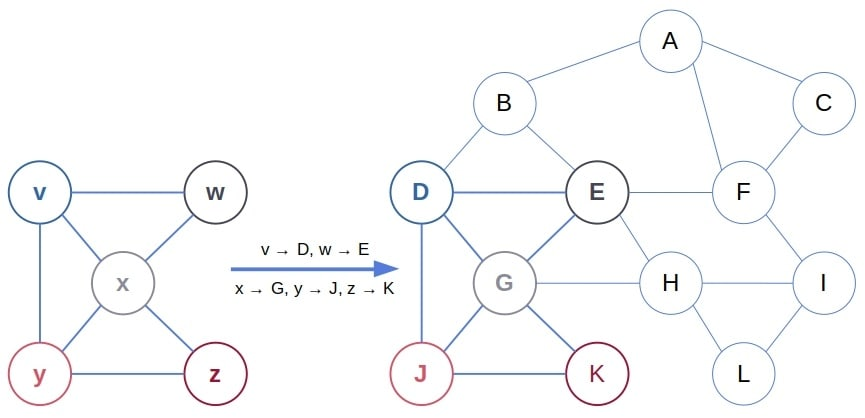## 1. Introduction

In this tutorial, we’ll explain what an induced subgraph is. We’ll also show how to construct it, how to check if a (sub)graph is induced, and how to find the induced graph isomorphism.

## 2. Subgraphs

Let’s say we have a graph, whereis the set of nodes, anddenotes the edges between them. A subgraph ofis any graphsuch thatand. In other words, each node in a subgraph is also a node in the supergraph. Further, every edge in the subgraph is an edge in the supergraph.

For example, all these graphs:Can be listed as subgraphs of:## 3. Induced Subgraphs

An induced subgraph is a special case of a subgraph. Ifis a subset of‘s nodes, then the subgraph ofinduced byis the graph that hasas its set of vertices and contains all the edges ofthat have both endpoints in. This definition covers both directed and undirected graphs. Also, it covers the weighted just as the unweighted ones.

Givenand, the induced subgraph is unique. There’s only one subgraph induced byin the above graph:### 3.1. What’s the Difference?

The concepts of an induced subgraph and an ordinary subgraph are very similar. The difference is that an induced subgraph includes all the edges that have both endpoints in the inducing set, whereas an ordinary subgraph may miss some.

So, an induced subgraph keeps both adjacency and non-adjacency of the inducing vertices, in contrast to an ordinary subgraph that preserves only non-adjacency.

## 4. Induced Subgraph Problems and Algorithms

Let’s introduce a few problems related to induced subgraphs.

### 4.1. Induced Subgraph Construction

Here, the input consists of the graphand the inducing set. Our goal is to construct the induced subgraph. We’ll do so by iterating over the edges inand keeping only those whose both endpoints are in:The time complexity depends on how we represent the graphs. Using linked lists, we traverse each edge incident to a node intwice, so the time complexity is. On the other hand, if we use matrices, we traverse the whole row ofentries for each of theinducing nodes. Therefore, the complexity will be.

### 4.2. How to Check if a Subgraph Is Induced

In this problem, we have a graphand its subgraph(soand). Our goal is to check ifis an induced subgraph of. To do so, we iterate over the edges incident toin. If we find an edge that isn’t in, we can conclude thatisn’t an induced subgraph because it doesn’t preserve adjacency. Otherwise, we conclude thatis induced:As before, the complexity depends on the way we represent the graphs.

We don’t need to check ifcontains an edge not in. That’s because of the way we defined the input. Since we know thatis a subgraph of,can’t contain an edge that’s not in.

### 4.3. How to Check if a Graph Is an Induced Subgraph

However, if we getas a graph of some nodes in, we’ll have to check both thatis a subgraph and that it is induced.### 4.4. Induced Subgraph Isomorphism

The Induced Subgraph Isomorphism (ISI) is an injective mapping from one graphto an induced subgraph of another,. So, we getandat the input and find an ISI mapping from the former to the latter.

Unlike in the previous two problems,anddon’t use the same labels for their nodes. So, we have to find a mapping that translatesto an induced subgraph of:Formally, we say thatis an ISI if and only if it satisfies:

 (1)The first condition states thatis an injective function. The second is that an ISI preserves adjacency, and the third is that it also keeps non-adjacency.

This problem is NP-hard, which means that no polynomial-time algorithm for solving it is known to date. We can treat it as a constraint-satisfaction problem (CSP) and solve it like we solve other CSPs. The Equation (1) lists all the constraints we need to define the CSP and feed it to the universal solver. Eachforwill correspond to a CSP variable, and each variable will have the whole setas its domain.

## 5. Conclusion

In this article, we talked about induced and ordinary subgraphs. We also presented a few common problems related to the former subgraphs. Those are the induced subgraph construction, verification, and isomorphism.